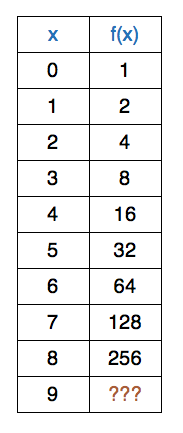Algebra

# Polynomial Interpolation: Level 5 Challenges

Consider the following system of linear equations:

$\begin{cases} \begin{array}{rcrcrcrcrcrcrl} 1&+&a&+&b&+&c&+&d&+&e&=&1\\ 32&+&16a&+&8b&+&4c&+&2d&+&e&=&2\\ 243&+&81a&+&27b&+&9c&+&3d&+&e&=&3\\ 1024&+&256a&+&64b&+&16c&+&4d&+&e&=&4\\ 3125&+&625a&+&125b&+&25c&+&5d&+&e&=&5&.\\ \end{array} \end{cases}$

Evaluate

$\left|\dfrac{8bcd}{125ae}\right|.$A polynomial $f(x)$ has degree $8$ and $f(i)=2^i$ for $i=0,1,2,3,4,5,6,7,8.$

Find $f(9).$

Let $a,b,c$ be complex numbers satisfying

$(a+1)(b+1)(c+1) = 1$

$(a+2)(b+2)(c+2) = 2$

$(a+3)(b+3)(c+3) = 3$

Find $(a+4)(b+4)(c+4)$.

Find the number of positive integers $n$ such that $2 \leq n \leq 100$ and there exists a polynomial $f(x)$ with real coefficients and degree $ such that for all integers $x,$ $f(x)$ is an integer if and only if $x$ is not a multiple of $n.$

Suppose $f(x)$ is a degree-$8$ polynomial such that $f(2^i)=\frac{1}{2^i}$ for all integers $0 \leq i \leq 8$. If $f(0)= \frac{a}{b}$, where $a$ and $b$ are coprime positive integers, what is the value of $a+b?$

×